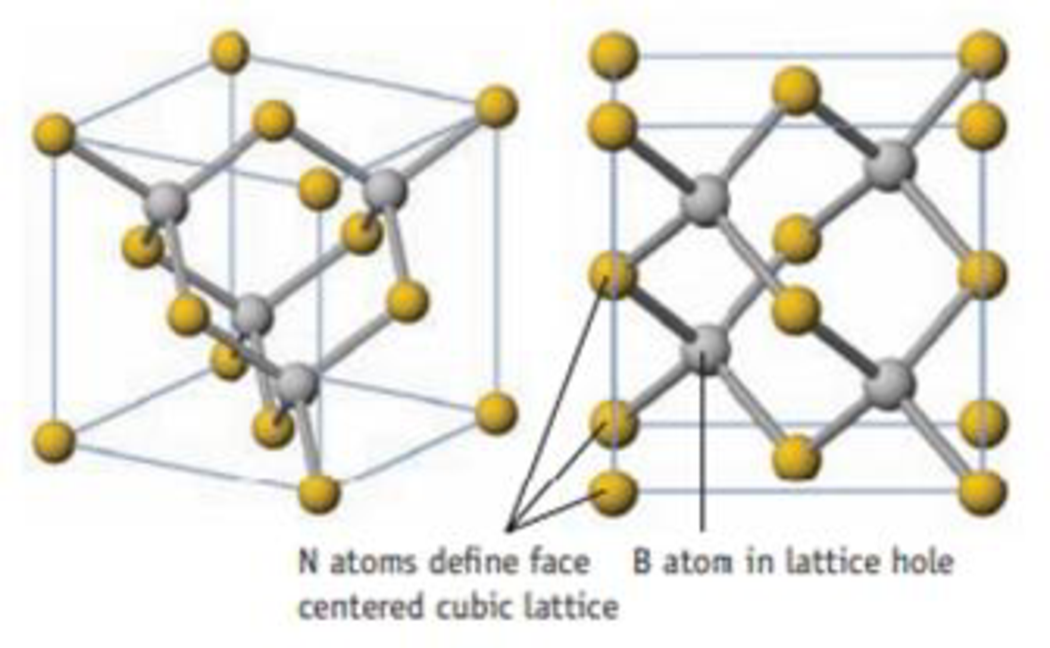Chapter 21, Problem 130SCQ

Chapter
Section
Textbook Problem

Boron nitride, BN, has the same solid state structure as ZnS (Figure 12.10).You can consider it a face-centered cube of nitrogen atoms with boron atoms in one half of the tetrahedral holes of the lattice. If the density of this form of BN is 3.45 g/cm3, what is the B–N bond length?

Interpretation Introduction

Interpretation:

To determine the BN bond length in the given cubic lattice.

Concept introduction:

There are two types of the cubic lattice of ZnS, zinc-blende and wurtzite. Zinc blende is based on a face-centred cubic lattice of anions and cations occupying one-half of the tetrahedral holes. Each ion is four coordinate and has local tetrahedral geometry.

If one constituent particles lie at the centre of each face and the particles lying at the centre is known as a face-centred cubic lattice.

The density of unit-cell is expressed as,

D=n×MNA×a3gcm3 (1)

Here,

The density of unit cell is denoted by D

The number of atoms is denoted by n

The molar mass of each atom in the unit-cell is denoted by M

The Avogadro number is denoted by NA

The bond-length is denoted by a

Bond length is the distance between the nuclei in a bond and it is related to the sum of the covalent radii at the bonded atoms.

Explanation

The BN bond length is calculated below.

Given:

The density of given unit-cell is 3.45gcm3.

The molar mass (M) of BN molecule is 24.82gmol1.

The value of Avogadro number NA is 6.022×1023mol1.

Total number of nitrogen (n) atoms per unit cell in a face-centered cubic lattice is,

n=18(8)+12(6)=4

Total number of boron (n) atoms per unit cell occupy one-half of the tetrahedral holes is,

n=12(8)=4

Therefore, the total number of atoms in a unit-cell is 4

Still sussing out bartleby?

Check out a sample textbook solution.

See a sample solution

The Solution to Your Study Problems

Bartleby provides explanations to thousands of textbook problems written by our experts, many with advanced degrees!

Get Started

Name the following molecular compounds a ClF4 b CS2 c PF3 d SF6

General Chemistry - Standalone book (MindTap Course List)

Chemistry In Focus

What observation led Edward Jenner to attempt his experiment?

Introduction to General, Organic and Biochemistry

Convert 4.50102nm into m. a.4.50102m b.4.501011m c.4.50107m d.4.50108m

Chemistry for Today: General, Organic, and Biochemistry